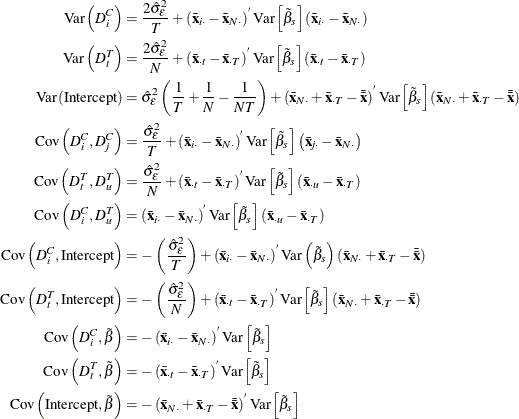# The PANEL Procedure

### Balanced Panels

Subsections:

Assume that the data are balanced (for example, all cross sections have T observations). Then you can write the following: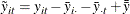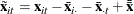where the symbols: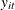and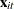are the dependent variable (a scalar) and the explanatory variables (a vector whose columns are the explanatory variables not including a constant), respectively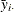and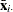are cross section means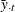and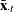are time means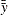and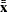are the overall means

The two-way fixed-effects model is simply a regression of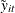on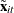. Therefore, the two-wayis given by: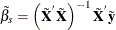The calculations of cross section dummy variables, time dummy variables, and intercepts follow in a fashion similar to that used in the one-way model.

First, you obtain the net cross-sectional and time effects. Denote the cross-sectional effects byand the time effects by. These effects are calculated from the following relations: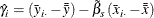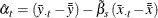Denote the cross-sectional dummy variables and time dummy variables with the superscript C and T. Under the NOINT option the following equations give the dummy variables: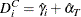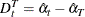When an intercept is specified, the equations for dummy variables and intercept are: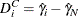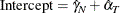The sum of squared errors is: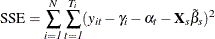The estimated error variance is: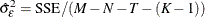With or without a constant, the variance covariance matrix of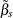is given by: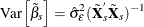#### Variance Covariance of Dummy Variables with No Intercept

The variances and covariances of the dummy variables are given with the NOINT specification as follows: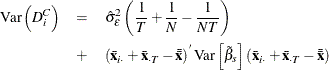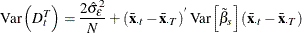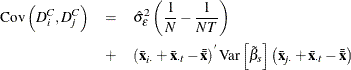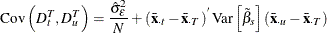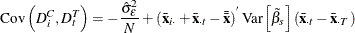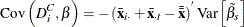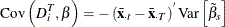#### Variance Covariance of Dummy Variables with Intercept

The variances and covariances of the dummy variables are given when the intercept is included as follows: